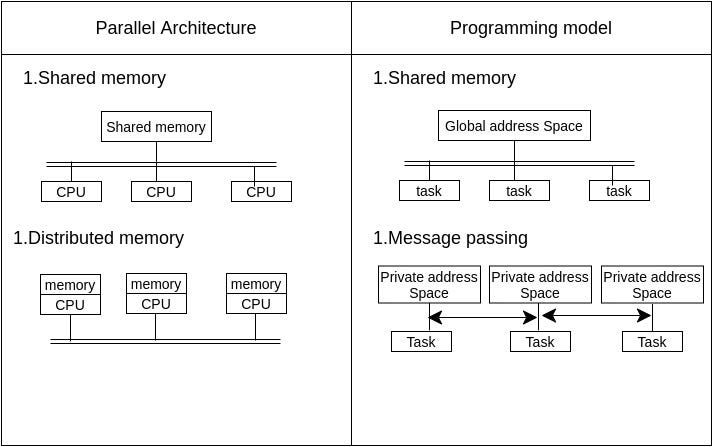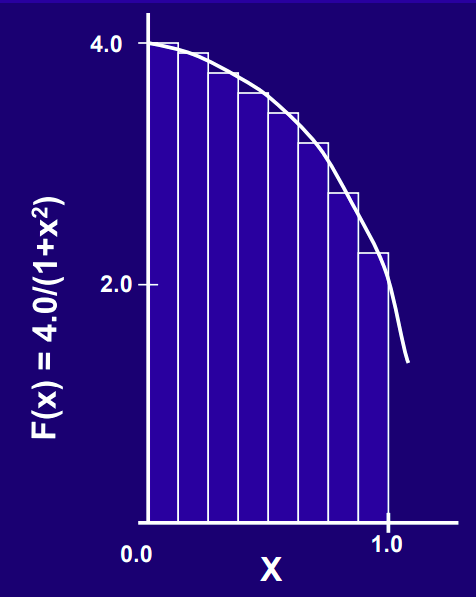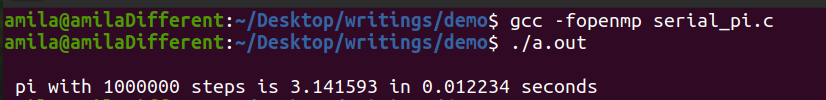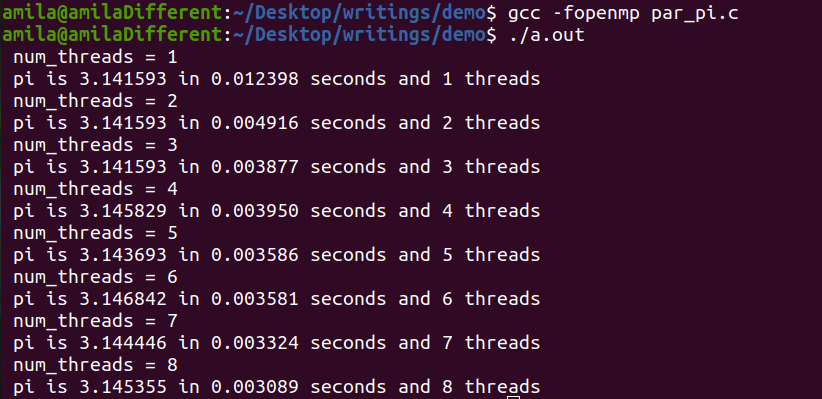# Step into Parallel computing

## Why do programmers need parallel computers?

Traditionally computer programs are written to perform serial computations. That is the algorithms can be taken as a set of sequential instructions, which will execute one after another in the CPU. When we go through the history of computers, as time grows the performance of computers increased. So programmers had no requirement to go out of serial computing because whatever they want to compute was facilitated by the better underlying hardware infrastructure which was improved drastically.

## Feel like we don't need parallel computing ??

Let’s see, as computer engineers or scientists we all know the “Moores's law” is associated with the complexity of computer chips ought to double roughly every two years. If you are more into electronics engineering you can take it as the number of transistors doubles and at the same time, the cost will be halved every year.

• Scientific computations
• Numerically intensive simulations
• Database operations and information systems
• AI /ML
• Deep learning
• Real-time systems and control applications

## What is parallel computing?

The simple answer is doing things simultaneously. Traditionally, in serial computing, a problem is divided into a set of instructions and performed sequentially. In contrast parallel computing the problem is divided into parts and can be executed simultaneously in different processors.

• Bit-level parallelism
• Instruction level parallelism
• Task level parallelism
• Super word-level parallelism

## Parallel architectures and parallel models

Now you know about what is parallel computing. Let’s see how it is performed. There are many programming languages, APIs, libraries available to perform parallel computing. As engineers before we begin with a particular platform, we will look into the architecture and models for parallel computing which is classified according to the level at which hardware supports parallelism. The following summarises the programming models available for different parallel architectures.Figure 03: Parallel architectures and programming models

## Demonstration

For the scope of this article, I will explain some OpenMP programming with a shared memory model.OpenMP provides a set of compiler directives and library routines for parallel application programmers. It is basically an API to enable multi-threads within your program. Using it simplifies writing multi-threaded programs in Fortran, C, and C++.

1. Explain a computationally extensive algorithm with the mathematics behind it
2. Write the serial C code for the computation
3. Make the code parallel using OpenMP
4. Compare the results

## 1. Computationally extensive algorithm with the mathematics behind it

We will write a simple program in C language to calculate the value of PI(π). We can approximate the value of PI as a Reimann sum. Here we can calculate the value of PI using the following definite integral.Figure 04: Approximate value of PI using sum of areas of rectangles

## 2. Write the serial C code

First, I will put this equation into a simple C code.Figure 05: Result of serial execution

## 3. Make the code parallel using OpenMP

Now I have a computationally intensive program and I will parallelize the computationally intensive part of the code using OpenMP. Then I will change the number of threads used and I will measure the total time for execution.

`#pragma omp parallel`
` #pragma omp single `
`#pragma omp for reduction `Figure 06: Result of parallel execution

## Conclusion

This article was aimed to introduce the importance of parallel computing. After discussing the architectures, an example was shown using the OpenMP. This can be used as a starting point and explore more in the world of parallel computing paradigms including GPGPU Programming using CUDA which is even more interesting.

--

--